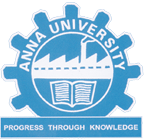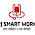MA8151 ENGINEERING MATHEMATICS 1 SYLLABUS - ANNA UNIVERSITY REGULATION 2017 - Anna University Internal marks 2018
OBJECTIVES :
The goal of this course is to achieve conceptual understanding and to retain the best traditions of traditional calculus. The syllabus is designed to provide the basic tools of calculus mainly for the purpose of modelling the engineering problems mathematically and obtaining solutions. This is a foundation course which mainly deals with topics such as single variable and multivariable calculus and plays an important role in the understanding of science, engineering, economics and computer science, among other disciplines.MA8151

UNIT I DIFFERENTIAL CALCULUS
Representation of functions - Limit of a function - Continuity - Derivatives - Differentiation rules - Maxima and Minima of functions of one variable.

UNIT II FUNCTIONS OF SEVERAL VARIABLES

Partial differentiation – Homogeneous functions and Euler‘s theorem – Total derivative – Change of variables – Jacobians – Partial differentiation of implicit functions – Taylor‘s series for functions of two variables – Maxima and minima of functions of two variables – Lagrange‘s method of undetermined multipliers.

UNIT III INTEGRAL CALCULUS
Definite and Indefinite integrals - Substitution rule - Techniques of Integration - Integration by parts, Trigonometric integrals, Trigonometric substitutions, Integration of rational functions by partial fraction, Integration of irrational functions - Improper integrals.

UNIT IV MULTIPLE INTEGRALS
Double integrals – Change of order of integration – Double integrals in polar coordinates – Area enclosed by plane curves – Triple integrals – Volume of solids – Change of variables in double and triple integrals.

UNIT V DIFFERENTIAL EQUATIONS
Higher order linear differential equations with constant coefficients - Method of variation of parameters – Homogenous equation of Euler‘s and Legendre‘s type – System of simultaneous linear differential equations with constant coefficients - Method of undetermined coefficients.

TOTAL: 60 PERIODS

OUTCOMES:

• After completing this course, students should demonstrate competency in the following skills:
• Use both the limit definition and rules of differentiation to differentiate functions.
• Apply differentiation to solve maxima and minima problems.
Evaluate integrals both by using Riemann sums and by using the Fundamental Theorem of Calculus.
• Apply integration to compute multiple integrals, area, volume, integrals in polar coordinates, in addition to change of order and change of variables.
• Evaluate integrals using techniques of integration, such as substitution, partial fractions and integration by parts.
• Determine convergence/divergence of improper integrals and evaluate convergent improper integrals.
• Apply various techniques in solving differential equations.

TEXT BOOKS :

1. Grewal B.S., ―Higher Engineering Mathematics‖, Khanna Publishers, New Delhi, 43rd Edition, 2014.
2. James Stewart, "Calculus: Early Transcendentals", Cengage Learning, 7th Edition, New Delhi, 2015. [For Units I & III - Sections 1.1, 2.2, 2.3, 2.5, 2.7(Tangents problems only), 2.8, 3.1 to 3.6, 3.11, 4.1, 4.3, 5.1(Area problems only), 5.2, 5.3, 5.4 (excluding net change theorem), 5.5, 7.1 - 7.4 and 7.8].

REFERENCES:

1. Anton, H, Bivens, I and Davis, S, "Calculus", Wiley, 10th Edition, 2016.
2. Jain R.K. and Iyengar S.R.K., ―Advanced Engineering Mathematics‖, Narosa Publications, New
Delhi, 3rd Edition, 2007.
3. Narayanan, S. and Manicavachagom Pillai, T. K., ―Calculus" Volume I and II,
S. Viswanathan Publishers Pvt. Ltd., Chennai, 2007.
4. Srimantha Pal and Bhunia, S.C, "Engineering Mathematics" Oxford University Press, 2015.
5. Weir, M.D and Joel Hass, "Thomas Calculus", 12th Edition, Pearson India, 2016.

1 comment:

1.Awesome post. It’s a great spot to find new information. Thank you for your positive post.

http://apjsmartworks.com/nokia-service-center-in-ambattur.html